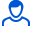Content: teorver_5582.doc (28.00 KB)

Positive responses: 0
Negative responses: 0

Refunds: 05582. x̅=113.20, n=64, σ=8. Find the confidence interval for estimating with a reliability of 0.99 the unknown mathematical expectation a of a normal distributed feature X of the general population, knowing the sample mean x̅=113.20, the sample size n=64 and the standard deviation σ=8.
Detailed solution. Decorated in Microsoft Word 2003 (Quest decided to use the formula editor)
No feedback yet